# MP Board Class 7th Maths Solutions Chapter 8 Comparing Quantities Ex 8.3

## MP Board Class 7th Maths Solutions Chapter 8 Comparing Quantities Ex 8.3

Question 1.
Tell what is the profit or loss in the following transactions. Also find profit percent or loss percent in each case.
(a) Gardening shears bought for ₹ 250 and sold for ₹ 325.
(b) A refrigerator bought for ₹ 12,000 and sold at ₹ 13,500.
(c) A cupboard bought for ₹ 2,500 and sold at ₹ 3,000.
(d) A skirt bought for ₹ 250 and sold at ₹ 150.
Solution:
(a) Cost price = ₹ 250,
Selling price = ₹ 325
Profit = 325 – 250 = ₹ 75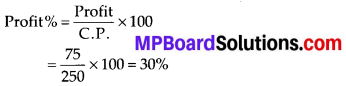(b) Cost price = ₹ 12000,
Selling price = ₹ 13500
Profit = 13500 – 12000 = ₹ 1500
Profit % = $$\frac{1500}{12000} \times 100=12.5 \%$$

(c) Cost price = ₹ 2500,
Selling price = ₹ 3000
Profit = 3000 – 2500 = ₹ 500(d) Cost price = ₹ 250,
Selling price = ₹ 150
Loss = 250 – 150 = ₹ 100Question 2.
Convert each part of the ratio to percentage:
(a) 3 : 1
(b) 2 : 3 : 5
(c) 1 : 4
(d) 1 : 2 : 5
Solution: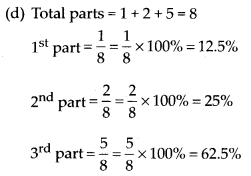Question 3.
The population of a city decreased from 25,000 to 24,500. Find the percentage decrease.
Solution:
Initial population = 25000 and Final population = 24500
Decrease = 500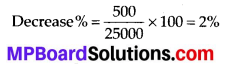Question 4.
Arun bought a car for ₹ 3,50,000. The next year, the price went upto ₹ 3,70,000. What was the percentage of price increase?
Solution:
Initial price = ₹ 350000
Final price = ₹ 370000
Increase = ₹ 20000Question 5.
I buy a T.V. for ₹ 10,000 and sell it at a profit of 20%. How much money do I get for it?
Solution:
Cost price = ₹ 10,000
Profit % = 20%
∴ Profit = 20% of 10000
Selling price = Profit + Cost price
$$=\frac{20}{100} \times 10000+10000$$
= 2000 + 10000 = ₹ 12,000

Question 6.
Juhi sells a washing machine for ₹ 13,500. She loses 20% in the bargain. What was the price at which she bought it?
Solution:
Selling price = ₹ 13500,
Loss% = 20%
Let the cost price be x.
∴ Loss = 20% of x
Cost price – Loss = Selling price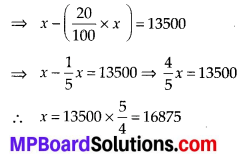Therefore, she bought it for ₹ 16875.Question 7.
(i) Chalk contains calcium, carbon and oxygen in the ratio 10 : 3 : 12. Find the percentage of carbon in chalk.
(ii) If in a stick of chalk, carbon is 3 g, what is the weight of the chalk stick?
Solution:
(i) Ratio of calcium, carbon and oxygen = 10 : 3 : 12
Therefore, percentage of carbon = $$\frac{3}{25} \times 100 \%$$
= 12%

(ii) Let the weight of the chalk stick be x g.Question 8.
Amina buys a book for ₹ 275 and sells it at a loss of 15%. How much does she sell it for?
Solution:
Cost price = ₹ 275
Loss% = 15% or Loss = 15% of 275
Cost price – Loss = Selling price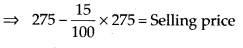⇒ 275 – 41.25 = Selling price
∴ Selling price = ₹ 233.75Question 9.
Find the amount to be paid at the end of 3 years in each case:
(a) Principal = ₹ 1,200 at 12% p.a.
(b) Principal = ₹ 7,500 at 5% p.a.
Solution:
(a) Principal (P) = ₹ 1200
Rate (R) = 12% p.a.
Time (T) = 3 years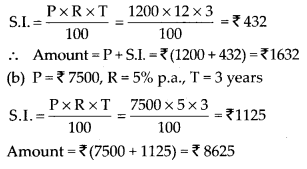Question 10.
What rate gives ₹ 280 as interest on a sum of ₹ 56,000 in 2 years?
Solution: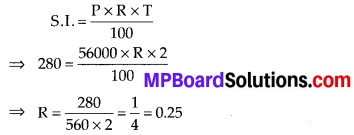Therefore, 0.25% gives ₹ 280 as interest on the given sum.Question 11.
If Meena gives an interest of ₹ 45 for one year at 9% rate p.a„ What is the sum she has borrowed?
Solution: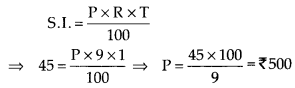Therefore, Meena has borrowed ₹ 500.